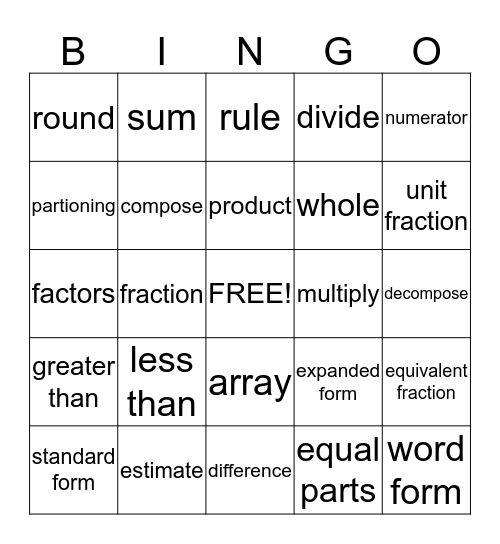# Math VocabularyThis bingo card has a free space and 24 words: less than, estimate, product, standard form, numerator, round, partioning, sum, multiply, array, whole, factors, expanded form, equivalent fraction, compose, equal parts, decompose, rule, unit fraction, fraction, word form, greater than, divide and difference.

## Play Online